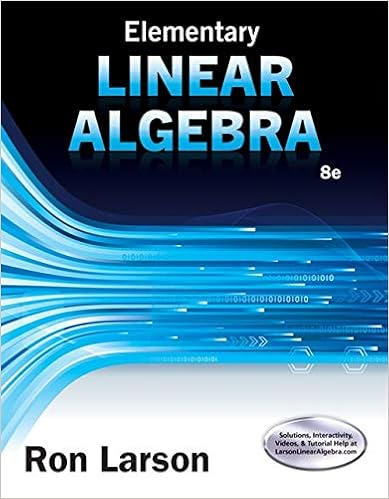# As a sum of two vectors a x and a y along the x and

• Lab Report
• 5
• 71% (24) 17 out of 24 people found this document helpful

This preview shows page 2 - 3 out of 5 pages.

##### We have textbook solutions for you!
The document you are viewing contains questions related to this textbook.The document you are viewing contains questions related to this textbook.
Chapter 4 / Exercise 60
Elementary Linear Algebra
LarsonExpert Verified
as a sum of two vectorsAxandAy along the x and the y-axis respectively, as shown.Axand Ayare calledthe componentsof vector A and are given byAx= A cosα Ay= A sinα α (≤ 900)is the angle vector Amakes with the x-axis.In order to find the resultant vector Rof a system of vectors A, B, C, etc we follow these steps:a) Find the x and y components for each vector using the above equations. i.e find:
y ....
b) Add up these components to get:
+ …
+ …
R is:
##### We have textbook solutions for you!
The document you are viewing contains questions related to this textbook.The document you are viewing contains questions related to this textbook.
Chapter 4 / Exercise 60
Elementary Linear Algebra
LarsonExpert Verified
•••